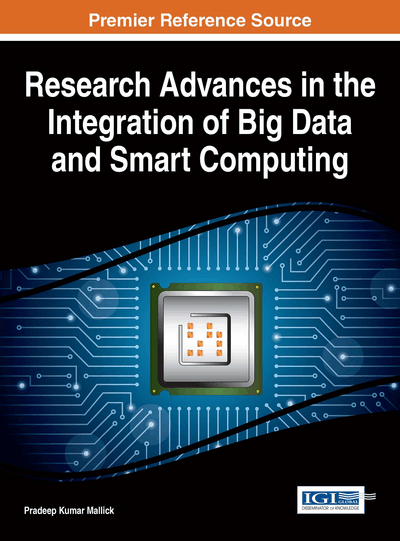# Signal Processing: Iteration Bound and Loop Bound

Deepika Ghai, Neelu Jain
DOI: 10.4018/978-1-4666-8737-0.ch009
OnDemand:
(Individual Chapters)
Available
\$29.50
No Current Special Offers

## Abstract

Digital signal processing algorithms are recursive in nature. These algorithms are explained by iterative data-flow graphs where nodes represent computations and edges represent communications. For all data-flow graphs, time taken to achieve output from the applied input is referred as iteration bound. In this chapter, two algorithms are used for computing the iteration bound i.e. Longest Path Matrix (LPM) and Minimum Cycle Mean (MCM). The iteration bound of single-rate data-flow graph (SRDFG) can be determined by considering the Multi-rate data-flow graph (MRDFG) equivalent of the SRDFG. SRDFG contain more nodes and edges as compared to MRDFG. Reduction of nodes and edges in MRDFG is used for faster determination of the iteration bound.
Chapter Preview
Top

## 1. Introduction

Iteration bound means time taken to achieve output from the applied input (Parhi & Messerschmitt, 1987). Many Digital Signal Processing (DSP) algorithms like recursive and adaptive digital filters contain feedback loops which induce an inherent lower bound on iteration period (Gerez, Heemstra & Herrmann, 1992). This bound is called iteration bound. It is a fundamental limit of recursive Data Flow Graph (DFG) which tells how fast DSP program can be executed in hardware (Lee, Chan & Verbauwhede, 2007). It is used as a characteristic in representation of algorithm in the form of DFG (Ito & Parhi, 1995).

A graphical representation of a DSP based system y(n) = ay(n – 1) + x(n) is shown in Figure 1(a). DFG representation of this equation is shown in Figure 1(b). It consists of a set of nodes and edges. The nodes represent computations and has an execution time associated with it (Lee & Messerschmitt, 1987). Node A represents addition and node B corresponds to multiplication. The node A and node B have an execution time of 4 u.t. (unit time) and 8 u.t. respectively. The edges represent communication between the nodes given by distinct direction. The edge A→B has zero delay and the edge B→A has one delay.

This chapter throws light on the following points:

• 1.

Defines critical path, loop bound and iteration bound.

• 2.

Algorithms such as Longest Path Matrix (LPM) and Minimum Cycle Mean (MCM) are defined to compute iteration bound.

• 3.

The iteration bound of Single-rate DFG (SRDFG) from Multi-rate DFG (MRDFG) is addressed.

### 1.1 Critical Path

It is the path of DFG with longest computation time but should not contain any delay. The speed of DSP system depends on the critical path computation time and it decreases with increase in computation time. Figure 2 shows DFG of a DSP system consisting: (1) loop, (2) path - with delay, and (3) path - without delay.

• 1. Loop

• 1→4→2→1

• 1→5→3→2→1

• 1→6→3→2→1

• 2.

Path - With Delay

• 1→4→2

• 1→5→3→2

• 1→6→3→2

• 3. Path - Without Delay

• 4→2→1

• 5→3→2→1

• 6→3→2→1

The maximum computation time (6 u.t.) with zero delay among these paths is 6→3→2→1. This path is known as critical path.

## Complete Chapter List

Search this Book:
Reset